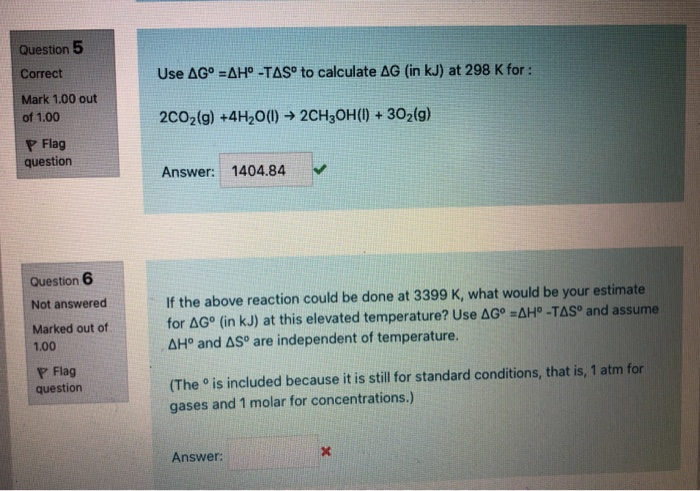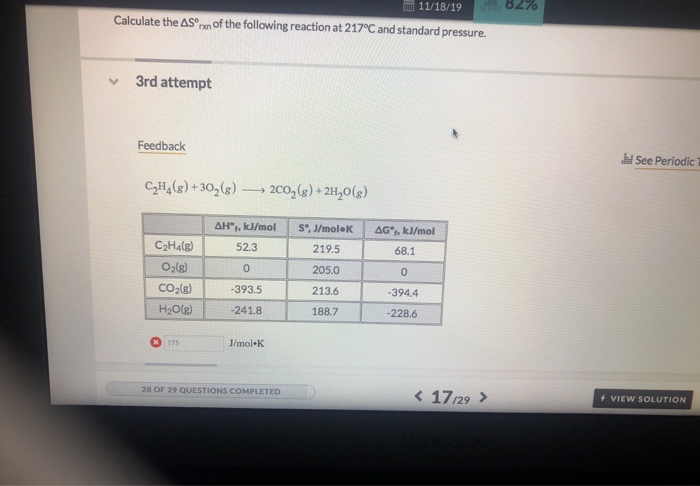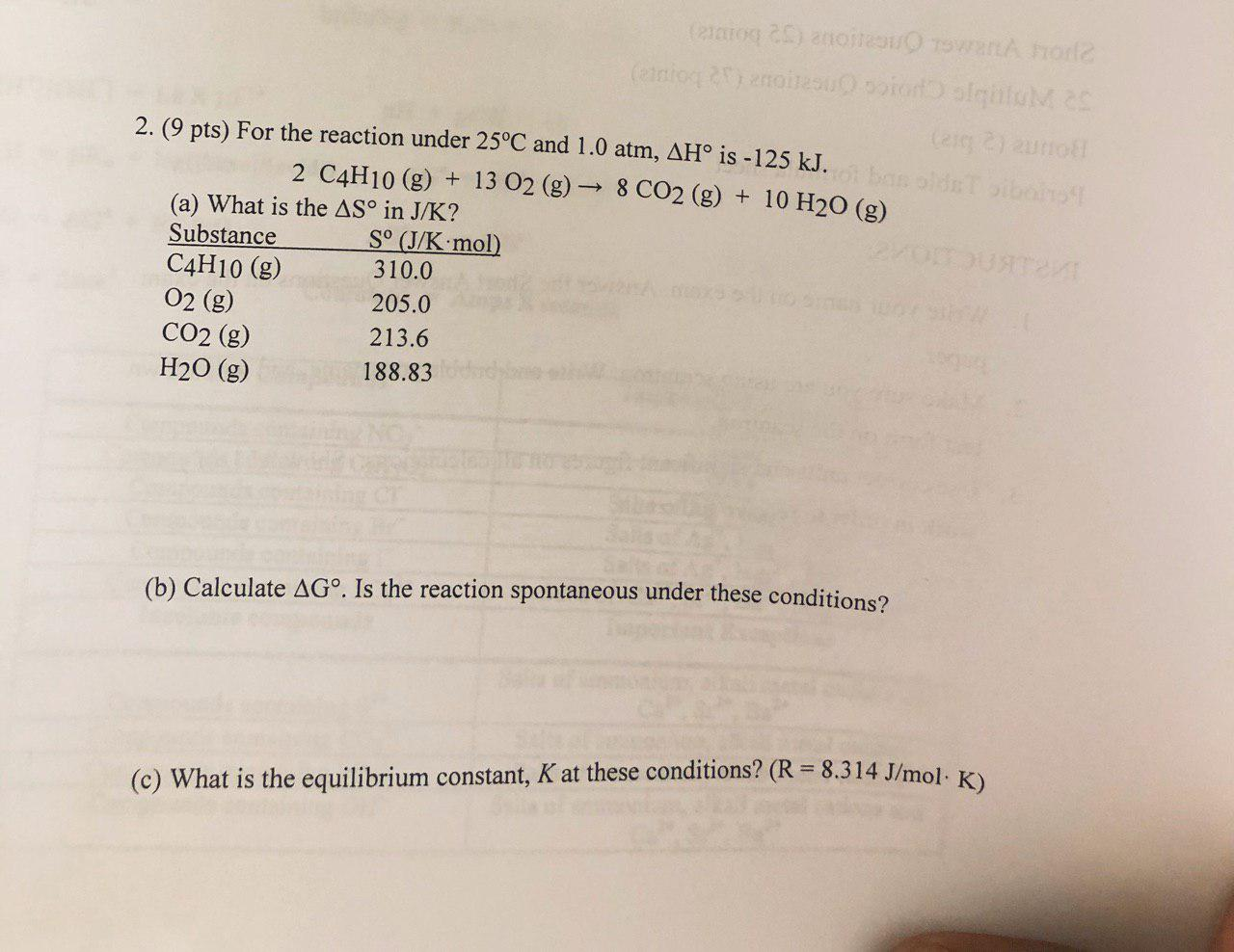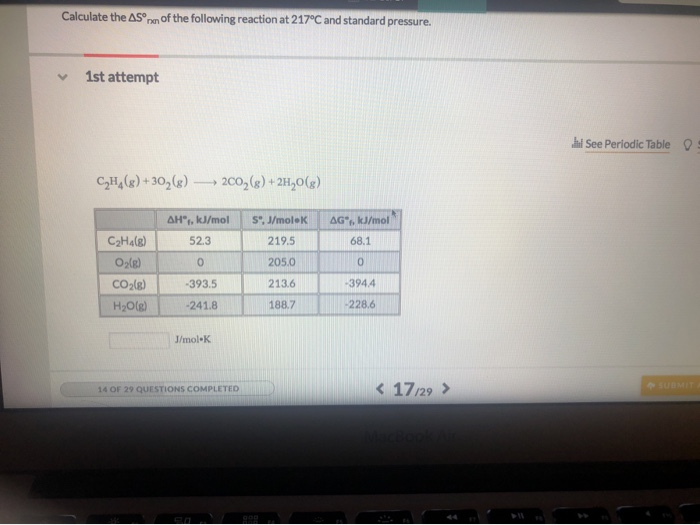Question

# (2 pts) Calculate the equilibrium pressure of CO2 in the system at 3000C. ΔH0 = 88.3...

(2 pts) Calculate the equilibrium pressure of CO2 in the system at 3000C.
ΔH0 = 88.3 kJ and ΔS0 = 151.3 J/K. R = 8.314 J/mol K
PbCO3(s) ® PbO(s) + CO2(g)
ΔH0f(kJ/mol) -699.1 -217.3 -393.5
S0(J/mol K) 131 68.7 213.6

T = 3000 + 273 = 3273 K

ΔGo = ΔHo - T ΔSo

= 88.3 - 3273 x 0.1513

= -406.9

ΔGo   = - R T ln K

-406.9 = -8.314 x 10^-3 x 3273 x ln K

ln K = 14.95

K = 3.11 x 10^6

K= PCO2

PCO2   = 3.11 x 10^6 atm

#### Earn Coins

Coins can be redeemed for fabulous gifts.

Similar Homework Help Questions
• ### Consider the reaction shown below. PbCO3(s) PbO(s) + CO2(g) Calculate the equilibrium pressure of CO2 in...

Consider the reaction shown below. PbCO3(s) PbO(s) + CO2(g) Calculate the equilibrium pressure of CO2 in the system at the following temperatures. (a) 300°C ____atm (b) 550°C _____atm Note: To find the value of the equilibrium constant at each temperature you must first find the value of G0 at each temperature by using the equation G0 = H0 - TS0 For this reaction the values are H0 = +88.3 kJ/mol and S0= 151.3 J/mol*K

• ### Consider the reaction shown below. PbCO3(s) PbO(s) + CO2(g) Calculate the equilibrium pressure of CO2 in...

Consider the reaction shown below. PbCO3(s) PbO(s) + CO2(g) Calculate the equilibrium pressure of CO2 in the system at the following temperatures. (a) 240°C ? atm (b) 600°C ? atm Note: To find the value of the equilibrium constant at each temperature you must first find the value of G0 at each temperature by using the equation G0 = H0 - TS0 For this reaction the values are H0 = +88.3 kJ/mol and S0= 151.3 J/mol*K

• ### What is ΔGo (in kJ) at 647 K for the following reaction? PbO(g) + CO2(g) →...

What is ΔGo (in kJ) at 647 K for the following reaction? PbO(g) + CO2(g) → PbCO3(s) PbO: ΔHfo = -219.0 kJ/mol and So = 66.5 J/K mol) PbCO3(s): ΔHfo = -699.1 kJ/mol and So = 131.0 J/K mol) CO2: ΔHfo = -393.5 kJ/mol and So = 213.6 J/K mol) At what temperature (in K) does the above reaction become spontaneous?

• ### 1.What is ΔGrxno (in kJ) at 440 K for the following reaction? PbO(g) + CO2(g) →...

1.What is ΔGrxno (in kJ) at 440 K for the following reaction? PbO(g) + CO2(g) → PbCO3(s) PbO: ΔHfo = -219.0 kJ/mol and So = 66.5 J/K mol) PbCO3(s): ΔHfo = -699.1 kJ/mol and So = 131.0 J/K mol) CO2: ΔHfo = -393.5 kJ/mol and So = 213.6 J/K mol) 2. At what temperature (in K) does the above reaction become spontaneous?

• ### 1-What is ΔGrxno (in kJ) at 338 K for the following reaction? PbO(g) + CO2(g) →...

1-What is ΔGrxno (in kJ) at 338 K for the following reaction? PbO(g) + CO2(g) → PbCO3(s) PbO: ΔHfo = -219.0 kJ/mol and So = 66.5 J/K mol) PbCO3(s): ΔHfo = -699.1 kJ/mol and So = 131.0 J/K mol) CO2: ΔHfo = -393.5 kJ/mol and So = 213.6 J/K mol) 2-At what temperature (in K) does the above reaction become spontaneous? PLEASE answer both

• ### Question 5 Correct Use AG° =AH° -TAS° to calculate AG (in kJ) at 298 K for...Question 5 Correct Use AG° =AH° -TAS° to calculate AG (in kJ) at 298 K for : Mark 1.00 out of 1.00 2002(g) +4H2O(1) ► 2CH3OH(1) + 302(g) P Flag question Answer: 1404.84 Question 6 Not answered Marked out of 1.00 If the above reaction could be done at 3399 K, what would be your estimate for AGº (in kJ) at this elevated temperature? Use AGⓇ =AH°-TAS and assume AH° and AS are independent of temperature. P Flag question (Theis...

• ### mm 11/18/19 su 82% Calculate the Sºn of the following reaction at 217°C and standard pressure....mm 11/18/19 su 82% Calculate the Sºn of the following reaction at 217°C and standard pressure. 3rd attempt Feedback See Periodic CH,(g) +302(8) 2002 (8) +24,0 (8) AH", kJ/mol 52.3 0 S", J/mol K 219.5 AG®, kJ/mol 68.1 205.0 0 C2H4(g) O2(8) CO2(g) H2O(g) -393.5 213.6 -394.4 -241.8 188.7 - 228.6 J/mol K 28 OF 29 QUESTIONS COMPLETED < 1729 > + VIEW SOLUTION

• ### (eao 20) anoiteu For 52 FDIDD:C CHo1ce Oneaou bopra eg 2) 2unot old:Toibolno 2. (9 pts)...(eao 20) anoiteu For 52 FDIDD:C CHo1ce Oneaou bopra eg 2) 2unot old:Toibolno 2. (9 pts) For the reaction under 25°C and 1.0 atm, AHo is -125 kJ. 2 C4H10 (g) 13 O2 (g) 8 CO2 (g) 10 H20 (g) (a) What is the AS° in J/K? Substance C4H10 (g) 02 (g) QURTRV So (J/K mol) 310.0 205.0 213.6 CO2 (g) 188.83 H20 (g) (b) Calculate AG°. Is the reaction spontaneous under these conditions? (c) What is the equilibrium constant,...

• ### Calculate the ASn of the following reaction at 217°C and standard pressure. 1st attempt lui See Periodic Table C2H,...Calculate the ASn of the following reaction at 217°C and standard pressure. 1st attempt lui See Periodic Table C2H,(8) +302(8) ► 2009 (8) + 2H,0(3) S",J/molek 219.5 AG®, l/mol 68.1 AH", IJ/mol CaHa(g) 52.3 O2(e) 10 CO2(8) -393.5 H2O(s) -241.8 205.0 213.6 -3944 188.7 228.6 J/mol. 14 OF 29 QUESTIONS COMPLETED < 17,29 >